# 5.2 Expression of Kcouple by a distance ratio factor

Analytical solutions can be obtained from (4.13) and  (4.14) for rectangular planes. This has been performed for power integrity analysis by  and . This work presents an analytical solution for a rectangular enclosure in Chapter 7. Mode decomposition requires numerical field simulation to extract the partial capacitances in the cross section of transmission lines on the PCB under the metallic enclosure cover. To enable a purely analytical solution for efficient design investigations, sources, traces, and planes are introduced into the cavity field by an analytical distance ratio factor. Complex PCBs usually consist of numerous traces and planes with different geometric shape, which also requires numerous simulations in a mode decomposition approach. Therefore, the efficiency of numerical algorithms for the solution of the cavity field inside enclosures can also be enhanced significantly by utilization of the proposed analytical introduction method.
The mode, considered in the cavity model, implies that the field does not vary in z-dimension. Therefore, the coupling factor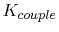can generally be expressed by a distance ratio weighting factor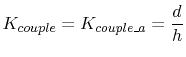(5.9)

According to Figure 5.1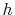denotes the vertical distance from the ground plane to the metallic enclosure cover and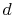is the vertical distance of the trace from the ground plane.

Table 5.1 compares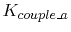from (5.9) to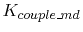from (5.7) for different trace and plane geometries. Values forwere obtained by numerical capacitance extraction with the Smart Analysis Program (SAP) from . A trace thickness of 35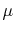m was used in the simulations, because this is often the copper layer thickness of PCBs. The difference between the two methods for the calculation of the coupling factoris smaller than three percent even for large trace distances to the ground plane, as in rows 4 and 5. The slight deviation can be explained by the trace thickness, which is considered in the numerical simulation, but not in (5.9). On a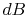scale, usually utilized to compare emission results, a deviation of three percent equals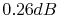. In comparison, the overall emission measurement uncertainty is usually larger than 3dB, even in very accurate laboratories. This measurement uncertainty considers, among other uncertainties, the antenna factor uncertainty, the antenna position tolerance, the site attenuation deviation, and the test receiver tolerances. Therefore, the accuracy of the analytical factor (5.9) is sufficient for EMC emission simulations.

Table 5.1: Comparison ofwith the distance ratio coupling factorfor several different plane/trace geometries. b is the trace width.
 Nr.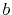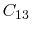difference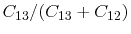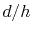mm mm mm F/m F/m % 1 2.0 7 0.20 113.81 3.46 0.02946 0.02857 -3.0 2 2.0 7 0.65 45.40 4.74 0.09445 0.09286 -1.7 3 2.0 7 1.50 25.15 6.94 0.21637 0.21429 -1.0 4 2.0 7 3.00 15.06 11.62 0.43550 0.42857 -1.6 5 0.1 7 0.65 16.76 1.76 0.09521 0.09286 -2.5 6 2.0 10 0.65 46.47 3.26 0.06555 0.06500 -0.8 7 2.0 15 0.65 47.39 2.18 0.04406 0.04333 -1.7 8 2.0 20 0.65 47.91 1.64 0.03305 0.03250 -1.7

C. Poschalko: The Simulation of Emission from Printed Circuit Boards under a Metallic Cover